Search by Topic

Resources tagged with Square numbers similar to Augustus' Age:

Filter by: Content type:
Age range:
Challenge level:

There are 24 results

Broad Topics > Numbers and the Number System > Square numbersAugustus' Age

Age 7 to 11 Challenge Level:

In 1871 a mathematician called Augustus De Morgan died. De Morgan made a puzzling statement about his age. Can you discover which year De Morgan was born in?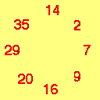Cycling Squares

Age 7 to 11 Challenge Level:

Can you make a cycle of pairs that add to make a square number using all the numbers in the box below, once and once only?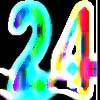Special 24

Age 7 to 11 Challenge Level:

Find another number that is one short of a square number and when you double it and add 1, the result is also a square number.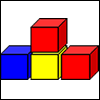Up and Down Staircases

Age 7 to 11 Challenge Level:

One block is needed to make an up-and-down staircase, with one step up and one step down. How many blocks would be needed to build an up-and-down staircase with 5 steps up and 5 steps down?A Right Charlie

Age 7 to 11 Challenge Level:

Can you use this information to work out Charlie's house number?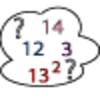Seven Square Numbers

Age 7 to 11 Challenge Level:

Add the sum of the squares of four numbers between 10 and 20 to the sum of the squares of three numbers less than 6 to make the square of another, larger, number.Sticky Numbers

Age 11 to 14 Challenge Level:

Can you arrange the numbers 1 to 17 in a row so that each adjacent pair adds up to a square number?One Wasn't Square

Age 7 to 11 Challenge Level:

Mrs Morgan, the class's teacher, pinned numbers onto the backs of three children. Use the information to find out what the three numbers were.Two Primes Make One Square

Age 7 to 11 Challenge Level:

Can you make square numbers by adding two prime numbers together?A Square Deal

Age 7 to 11 Challenge Level:

Complete the magic square using the numbers 1 to 25 once each. Each row, column and diagonal adds up to 65.Always, Sometimes or Never? Number

Age 7 to 11 Challenge Level:

Are these statements always true, sometimes true or never true?Making Boxes

Age 7 to 11 Challenge Level:

Cut differently-sized square corners from a square piece of paper to make boxes without lids. Do they all have the same volume?Odd Squares

Age 7 to 11 Challenge Level:

Think of a number, square it and subtract your starting number. Is the number you’re left with odd or even? How do the images help to explain this?Swimming Pool Tiles

Age 7 to 11 Challenge Level:

This activity creates an opportunity to explore all kinds of number-related patterns.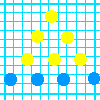Graphing Number Patterns

Age 7 to 11 Challenge Level:

Does a graph of the triangular numbers cross a graph of the six times table? If so, where? Will a graph of the square numbers cross the times table too?Digat

Age 11 to 14 Challenge Level:

What is the value of the digit A in the sum below: [3(230 + A)]^2 = 49280AFractions in a Box

Age 7 to 11 Challenge Level:

The discs for this game are kept in a flat square box with a square hole for each. Use the information to find out how many discs of each colour there are in the box.Picture a Pyramid ...

Age 7 to 11 Challenge Level:

Imagine a pyramid which is built in square layers of small cubes. If we number the cubes from the top, starting with 1, can you picture which cubes are directly below this first cube?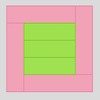Cuisenaire Rods

Age 7 to 11 Challenge Level:

These squares have been made from Cuisenaire rods. Can you describe the pattern? What would the next square look like?Square Routes

Age 11 to 14 Challenge Level:

How many four digit square numbers are composed of even numerals? What four digit square numbers can be reversed and become the square of another number?Time of Birth

Age 11 to 14 Challenge Level:

A woman was born in a year that was a square number, lived a square number of years and died in a year that was also a square number. When was she born?Light the Lights Again

Age 7 to 11 Challenge Level:

Each light in this interactivity turns on according to a rule. What happens when you enter different numbers? Can you find the smallest number that lights up all four lights?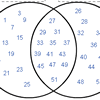Various Venns

Age 7 to 11 Challenge Level:

Use the interactivities to complete these Venn diagrams.Factors and Multiples Puzzle

Age 11 to 14 Challenge Level:

Using your knowledge of the properties of numbers, can you fill all the squares on the board?